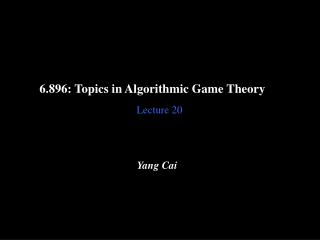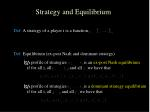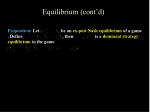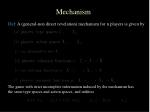Download Presentation6.896: Topics in Algorithmic Game Theory

# 6.896: Topics in Algorithmic Game Theory - PowerPoint PPT Presentation

6.896: Topics in Algorithmic Game Theory. Lecture 20. Yang Cai. Recap. Games with Strict Incomplete Information. Def : A game with (independent private values and) strict incomplete information for a set of n players is given by the following ingredients:. ( i ). (ii). (iii).I am the owner, or an agent authorized to act on behalf of the owner, of the copyrighted work described.
Download Presentation## 6.896: Topics in Algorithmic Game Theory

An Image/Link below is provided (as is) to download presentation

Download Policy: Content on the Website is provided to you AS IS for your information and personal use and may not be sold / licensed / shared on other websites without getting consent from its author.While downloading, if for some reason you are not able to download a presentation, the publisher may have deleted the file from their server.

- - - - - - - - - - - - - - - - - - - - - - - - - - E N D - - - - - - - - - - - - - - - - - - - - - - - - - -
Presentation TranscriptGames with Strict Incomplete Information

Def: A game with (independent private values and) strict incomplete information for a set of n players is given by the following ingredients:

(i)

(ii)

(iii)Strategy and Equilibrium

Def: A strategy of a player i is a function

Def: Equilibrium (ex-post Nash and dominant strategy)

• A profile of strategies is an ex-post Nash equilibrium
• if for all i, all , and all we have that
• A profile of strategies is a dominant strategy equilibrium
• if for all i, all , and all we have thatEquilibrium (cont’d)

Proposition: Let be an ex-post Nash equilibrium of a game . Define , then is a dominant strategy equilibrium in the game

.General Mechanisms

Vickrey’s auction and VCG are both single round and direct-revelation mechanisms.

We will give a general model of mechanisms. It can model multi-round and indirect-revelation mechanisms.Mechanism

Def: A (general-non direct revelation) mechanism for n players is given by

The game with strict incomplete information induced by the mechanism has the same type spaces and action spaces, and utilitiesImplementing a social choice function

Given a social choice function

A mechanism implements in dominant strategies if for some dominant strategy equilibrium of the induced game, we have that for all

, .

Ex: Vickrey’s auction implements the maximum social welfare function in dominant strategies, because is a dominant strategy equilibrium, and maximum social welfare is achieved at this equilibrium.

Similarly we can define ex-post Nash implementation.

outcome of the social choice function

outcome of the mechanism at the equilibrium

Remark: We only requires that for some equilibrium and allows other equilibria to exist.Revelation Principle

We have defined direct revelation mechanisms in previous lectures. Clearly, the general definition of mechanisms is a superset of the direct revelation mechanisms.

But is it strictly more powerful? Can it implement some social choice functions in dominant strategy that the incentive compatible (direct revelation dominant strategy implementation) mechanism can not?Revelation Principle

Proposition: (Revelation principle) If there exists an arbitrary mechanism that implements in dominant strategies, then there exists an incentive compatible mechanism that implements . The payments of the players in the incentive compatible mechanism are identical to those, obtained at equilibrium, of the original mechanism.IncentiveCompatible

Def: A mechanism is called incentive compatible, or truthful , or strategy-proofiff for all i, for all and for all

utility of i if he says the truth

utility of i if he lies

i.e. no incentive to lie!Revelation Principle

Proposition: (Revelation principle) If there exists an arbitrary mechanism that implements in dominant strategies, then there exists an incentive compatible mechanism that implements . The payments of the players in the incentive compatible mechanism are identical to those, obtained at equilibrium, of the original mechanism.

Proof idea: SimulationRevelation Principle (cont’d)

new mechanism

original mechanismProof of Revelation Principle

Proof: Let be a dominant strategy equilibrium of the original mechanism such that , we define a new direct revelation mechanism:

Since each is a dominant strategy for player i, for every , we have that

Thus in particular this is true for all and any we have that

which gives the definition of the incentive compatibility of the mechanism.Revelation Principle (cont’d)

Corollary: If there exists an arbitrary mechanism that ex-post Nash equilibrium implements , then there exists an incentive compatible mechanism that implements . Moreover, the payments of the players in the incentive compatible mechanism are identical to those, obtained in equilibrium, of the original mechanism.

Proof sketch: Restrict the action spaces of each player. By the previous proposition, we know in the restricted action spaces, the mechanism implements the social choice function in dominant strategies. Now we can invoke the revelation principle to get an incentive compatible mechanism.Characterizations

What social choice functions can be implemented?

• Only look at incentive compatible mechanisms (revelation principle)
• When is a mechanism incentive compatible?
• Characterizations of incentive compatible mechanisms.
• Maximization of social welfare can be implemented (VCG). Any others?
• Basic characterization of implementable social choice functions.Direct Characterization

A mechanism is incentive compatible iff it satisfies the following conditions for every i and every :

(i)

i.e., for every , there exists a price , when the chosen alternative is , the price is

(ii)

i.e., for every , we have alternative where the quantification is over all alternatives in the range ofDirect Characterization (cont’d)

Proof:

(if part) Denote

and . Since the mechanism optimizes for i, the utility of i when telling the truth is not less than the utility when lying.Direct Characterization (cont’d)

Proof (cont):

(only if part; (i)) If for some , but

. WLOG, we assume

. Then a player with

type will increase his utility by declaring .

(only if part; (ii)) If , we fix

and

Now a player with type will increase his

utility by declaring .Weak Monotonicity
• The direct characterization involves both the social choice function and the payment functions.
• Weak Monotonicityprovides a partial characterization that only involves the social choice function.Weak Monotonicity (WMON)

Def: A social choice function satisfies Weak Monotonicity (WMON) if for all i, all we have that

i.e. WMON means that if the social choice changes when a single player changes his valuation, then it must be because the player increased his value of the new choice relative to his value of the old choice.Weak Monotonicity

Theorem: If a mechanism is incentive compatible then satisfies WMON. If all domains of preferences are convex sets (as subsets of an Euclidean space) then for every social choice function that satisfies WMON there exists payment function such that

is incentive compatible.

Remarks: (i) We will prove the first part of the theorem. The second part is quite involved, and will not be given here.

(ii) It is known that WMON is not a sufficient condition for incentive compatibility in general non-convex domains.Weak Monotonicity (cont’d)

Proof: (First part) Assume first that is incentive compatible, and fix i and in an arbitrary manner. The direct characterization implies the existence of fixed prices for all

(that do not depend on ) such that whenever the outcome is then i pays exactly .

Assume . Since the mechanism is incentive compatible, we have

Thus, we haveMinimization of Social Welfare

We know maximization of social welfare function can be implemented.

How about minimization of social welfare function?

No! Because of WMON.Minimization of Social Welfare

Assume there is a single good. WLOG, let . In this case, player 1 wins the good.

If we change to , such that . Then player 2 wins the good. Now we can apply the WMON.

The outcome changes when we change player 1’s value. But according to WMON, it should be the case that . But . Contradiction.Weak Monotonicity

WMON is a good characterization of implementable social choice functions, but is a local one.

Is there a global characterization?Affine Maximizer

Def: A social choice function is called an affine maximizer if for some subrange , for some weights and for some outcome weights , for every , we have thatPayments for Affine Maximizer

Proposition: Let be an affine maximizer. Define for every i,

where is an arbitrary function that does not depend on . Then, is incentive compatible.Payments for Affine Maximizer

Proof: First, we can assume wlog . The utility of player i if alternative is chosen is . By multiplying by this expression is maximized when

is maximized which is what happens when i reports truthfully.Roberts Theorem

Theorem [Roberts 79]: If , is onto , for every i, and

is incentive compatible then is an affine maximizer.

Remark: The restriction is crucial (as in Arrow’s theorem), for the case , there do exists incentive compatible mechanisms beyond VCG.